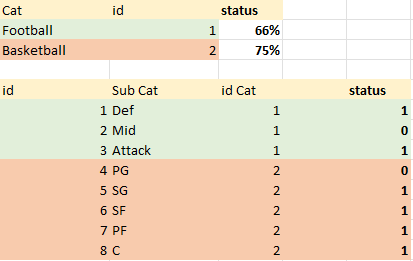cancel
Showing results for
Did you mean:Frequent Visitor

## extract percentage from true/false column

Hi all,

I have true/false column data and I want to calculate percentage of those true/false values from subcategory to category.

Here is example of the data.I want to calculate status column

Please let me know if you have any advice how to do it

1 ACCEPTED SOLUTIONSuper User

Hi @pnem ,

You can create a column as below:-

``````STATUS =
var _true = COUNTROWS(FILTER('Table 2','Table 1'[ID]='Table 2'[ID CAT] && 'Table 2'[STATUS] = 1))
var _total = COUNTROWS(FILTER('Table 2','Table 1'[ID]='Table 2'[ID CAT]))
return DIVIDE(_true,_total,0)``````

Refer a file below:-

Best Regards,
Samarth

If this post helps, please consider accepting it as the solution to help the other members find it more quickly.Super User

Hi @pnem ,

You can create a column as below:-

``````STATUS =
var _true = COUNTROWS(FILTER('Table 2','Table 1'[ID]='Table 2'[ID CAT] && 'Table 2'[STATUS] = 1))
var _total = COUNTROWS(FILTER('Table 2','Table 1'[ID]='Table 2'[ID CAT]))
return DIVIDE(_true,_total,0)``````

Refer a file below:-

Best Regards,
Samarth

If this post helps, please consider accepting it as the solution to help the other members find it more quickly.